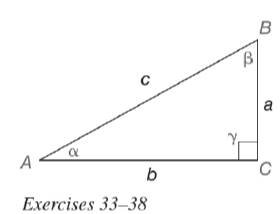Chapter 11.3, Problem 33EElementary Geometry For College St...

7th Edition
Alexander + 2 others
ISBN: 9781337614085

Solutions

Chapter
SectionElementary Geometry For College St...

7th Edition
Alexander + 2 others
ISBN: 9781337614085
Textbook Problem

In Exercises 33 to 38, we expand the list of trigonometric identities. As you may recall, an identity is a statement that is true for all permissible choices of the variable (see page 510.)a) For α ≠ 90 ∘ , prove the identity tan α = sin α cos α (HINT: sin α = a c and cos α = b c . )b) Use your calculator to show that tan 23 ∘ and sin 23 ∘ cos 23 ∘ are equivalent.To determine

(a)

To prove:

For α90, the identity tanα=sinαcosα.

Explanation

Given:

For α90, tanα=sinαcosα.

Definition used:

The trigonometric ratios:

Calculation:

Consider the angle α.

From the figure, the length of the opposite leg is a, the length of the adjacent leg is b and the hypotenuse is c.

sinα=ac

cosα=bc

tanα=ab   ...(1)

Consider sinαcosα.

To determine

(b)

To show:

The trigonometric ratio tan23 is equivalent to sin23cos23 using calculator.

Still sussing out bartleby?

Check out a sample textbook solution.

See a sample solution

The Solution to Your Study Problems

Bartleby provides explanations to thousands of textbook problems written by our experts, many with advanced degrees!

Get Started

Evaluate the integrals in Problems 5-28. Check your answers by differentiating. 7.

Mathematical Applications for the Management, Life, and Social Sciences

Evaluate the integral. xtan2xdx

Calculus (MindTap Course List)

In Exercises 11-14, factor the expression. x23x+2

Calculus: An Applied Approach (MindTap Course List)

Evaluate dx using the Fundamental Theorem of Calculus. 6 12 18 24

Study Guide for Stewart's Single Variable Calculus: Early Transcendentals, 8th

In Exercises 15-28, construct a truth table for the compound proposition. (pq)

Finite Mathematics for the Managerial, Life, and Social Sciences